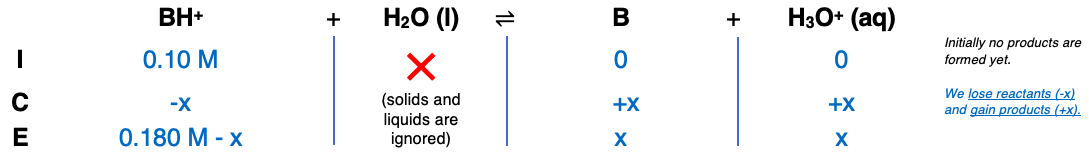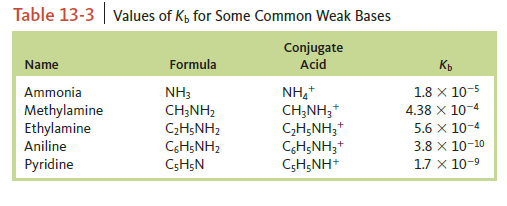# Problem: Consider a solution of an unknown salt having the general formula BHCl, where B is one of the weak bases in the following table. A 0.10-M solution of the unknown salt has a pH of 5.82. What is the actual formula of the salt?

###### FREE Expert Solution

Step 1Step 2

[H3O+] = x = 1.5136x10-6 M

Step 3

[BH+] = 0.180 M - 1.5136x10-6 M = 0.180 M

[B] = [H3O+] = 1.5136x10-6 M###### Problem Details

Consider a solution of an unknown salt having the general formula BHCl, where B is one of the weak bases in the following table. A 0.10-M solution of the unknown salt has a pH of 5.82. What is the actual formula of the salt?